## Wednesday, June 24, 2020

### Baudhayana: The man who knew Pythagoras theorem 300 years before him

Baudhayana is believed to have born around 800 BCE while Pythagoras was born in 570 BCE. The popular theorem that carries the name of Pythagoras says square of length of hypotenuse  is equal to sum of squares of two other sides in  a right angle triangle. While not said in so many words, here is what Boudhayana says in his book Sulba Sutra.

चतुरश्राच्चतुरश्रं निर्जिहीर्षन्यावन्निर्जिहीर्षेत्तस्य करण्या वर्षीयसो वृघ्रमुल्लिखेहृघ्रस्य
पार्श्वमानीमक्ष्णयेतरत्पार्श्वमुपसहंरेत्सा यत्र निपतेत्तदपच्छिन्धाच्छिन्नया निरस्तम्

If you wish to deduct one square from another use the following method.
1. Cut off from the larger one an oblong with the side of the smaller one
2. Draw one of the sides of that oblong across to the other side
3. Where it touches the other side, that piece cut off; but it the deduction is made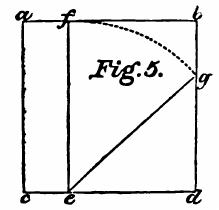Look at the figure above, abcd is the larger square, we want to cut from it a square of length of the side de. Draw an arc of length ef from f to g. Now dg is the length of the square which is arrived after subtracting from square abcd and square of length de.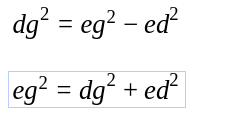This is nothing but what is also known as Pythagoras theorem.

## Monday, June 22, 2020

### Aryabhatta and Eclipses

Yesterday was a solar eclipse and the usual superstitions about eclipses were doing the rounds of internet. Here is what Aryabhatta had figured out in 400-500AD about eclipses.

स्फुटशशिमासान्तेऽर्क पातासन्नो यदा प्रविशतीन्दुः |
भूच्छायाँ  पक्षान्ते तदाधिकोनं ग्रहणमध्यम् ॥
At the end of the lunar month, the Moon, lying near a node (of the moon), enters the Sun, or, at the end of lunar fortnight, enters the Earth's Shadow, it is more or less the middle of an eclipse. (Solar eclipse in the format case and lunar eclipse in the latter case).

भूरविविवरं विभजेद् भूगुणितं तु रविभूविशेषेण|
भूच्छायादीर्घत्वं लब्धं भूगोलविष्कम्भात्  ॥
Multiply the distance of the Sun from the Earth by the diameter of the Earth and divide the product by the difference between the diameters of the Sun and the Earth : the result is the length of the shadow of the Earth (i.e. the distance of the vertex from the Earth's shadow) from the diameter of the Earth (i.e. from the center of the Earth)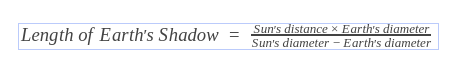The old Hindu method of deriving this formula, called the The Lamp and Shadow method, (प्रदिपच्छ्यकर्म) is as follows.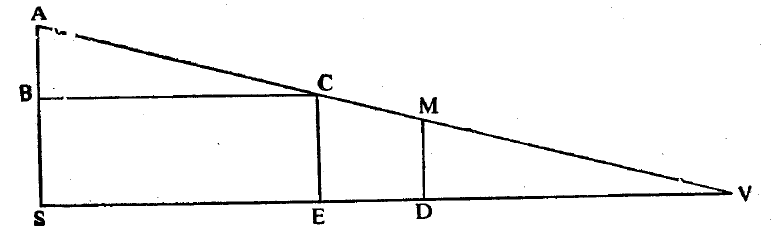In the figure above, S is the center of the Sun and E is the center of Earth. SA and EC are drawn perpendicular to SE and denote the semi-diameter of the Sun and Earth respectively. BC is parallel to SE. V is the point where SE and AC produced meet each other. If SA is considered to be a lamp post, EC is a gnomon and EV is the shadow cast by the gnomon due to the light of the lamp. Consequently EV is the length of Earth's shadow from the diameter of the earth.
The triangles CEV and ABC are similar.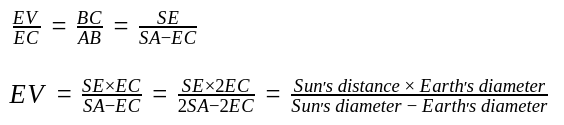Aryabhatta extensively studied phenomenon like eclipses and described various details about  it in his book. Looks like superstitious crowd is none the wiser after 1500 years.

## Wednesday, June 17, 2020

### Squarerooting by Aryabhatta

Here is the squareroot method of Aryabhatta.
भागं हरेदवर्गान्नित्यं द्विगुणेन वर्गमूलेन |
वर्गाद्वर्गे शुद्धे लब्धं स्थानान्तरे मूलम् ||
What above rule implies is, (Having subtracted the greatest possible square from the last odd place and then having written down the square root of the number subtracted in the line of square root), always divide the even place (standing on the right) by twice the square root. Then, having subtracted the square (of the quotient) from the odd place (standing on the right), set down the quotient at the next place (i.e., on the right of the number already written in the line of the square root). This is the square root. (Repeat the process if there are still digits on the right).

In many of the Aryabhatta principles we see even and odd digits. These are the digits of the number counted from left to right with left most being 1. Let's take a number like 85137529 and try to apply above method.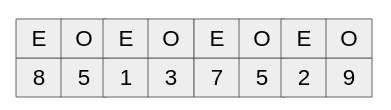The last odd place is 5 but since it is not the last digit, we take last two digits to start the computation. The process in simpler English is as follows.
1. Subtract the greatest possible from the last odd place
2. Divide the even place by twice the square root of the preceding old place
3. Subtract  the square of the quotient from the odd place
4. Repeat the process till we have more digits.

Since the left most digit is a even digit, we start with last two digit.
1. The largest square smaller than that is 81, so quotient is 9. Once we subtract 81 from 85, we get 4
2. Now we bring down 1 and divide the number from twice the quotient, dividing 41 from 18 gets us 36 with quotient of 2.
3. On subtracting 36 from 41 we get 5 and bringing down 3 gives us 53.
4. Now we subtract square of last quotient from this which gives us 53-4 = 49.
5. Now we bring down 7 and divide it by twice the quotient till now which is 92*2 = 184. Dividing 497 from 184 gives us 368 with quotient 2.
6. The subtraction gives us 129, we bring down 5 and subtract 4 which is the square of last quotient.
7. We get 1291, bring down 2 and divide it with twice the current quotient which 922 *2 = 1844 giving us a quotient 7.
8. We get 4, we bring down 9. This gives us 49,  Once we divide 49 from square of last quotient, we get zero and we have no more digits left. This (9227) is our square root.

## Monday, June 15, 2020

### Aryabhata, When was he born?

Aryabhata, the author of Aryabhatiya is sometimes confused with his namesake of the tenth century AD, the author of Maha-siddhanta (महा सिद्धांत). The author of Aryabhatiya is called Aryabhata I. In stanza 1 of chapter ii of the Aryabhatiya, he writes :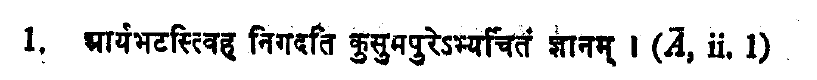Aryabhata  sets forth here the knowledge honoured at Kusumapura.
Many other commentators Paramesvara (AD 1431), Raghunatha-raja (AD 1597), and persian scholar Al-Biruni (AD 973-1048) confirm the fact that he was Aryabhata of Kusumapura. Bhaskara I (AD 629), the earliest commentator of Aryabhatiya, refers to Aryabhata I as Asamaka, his Aryabhatiya by the names Asmaka-tantra and Asmakiya, and identifies Kusumapura with Pataliputra in ancient Magadha.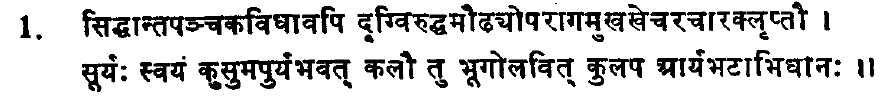When the methods of the five Siddhanta began to yield results conflicting with the observed phenomena such as the settings of the planets and the eclipses, etc, there appeared in the Kali age at Kusumapuri, Surya himself in the guise of Aryabhata, the Kulapa well versed in astronomy.
There is a verse in Aryabhatiya which  runs as follows:
When sixty times sixty years and three quarter-yugas had elapsed (of the current yuga), twenty-three years had then passed since by birth. This shows that Kali year 3600 elapsed and at that time Aryabhata was twenty seven years old. Kali year 3600 corresponds to AD 499, that implies that Aryabhata was born in year AD 476, incidentally that is the same year Buddhagupta took over the reigns of government at Pataliputra.
The Bihar Research Society, Patna, celebrates the birth anniversary of Aryabhata on April 13.